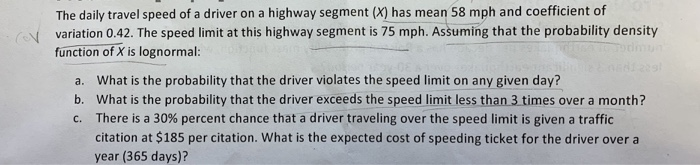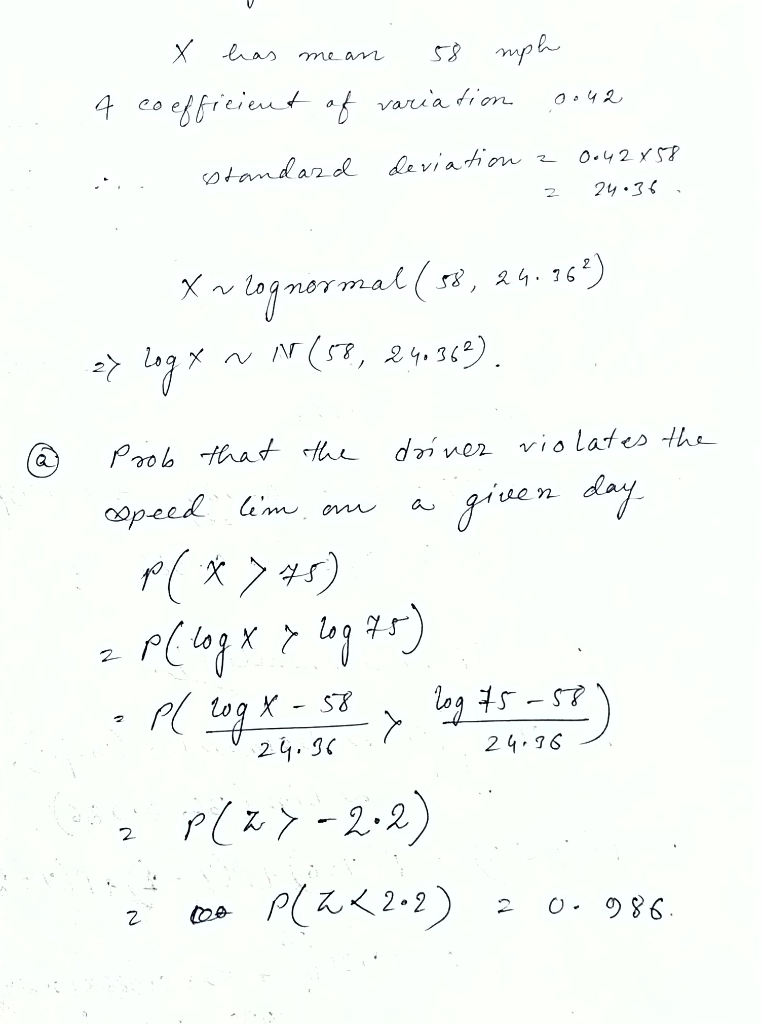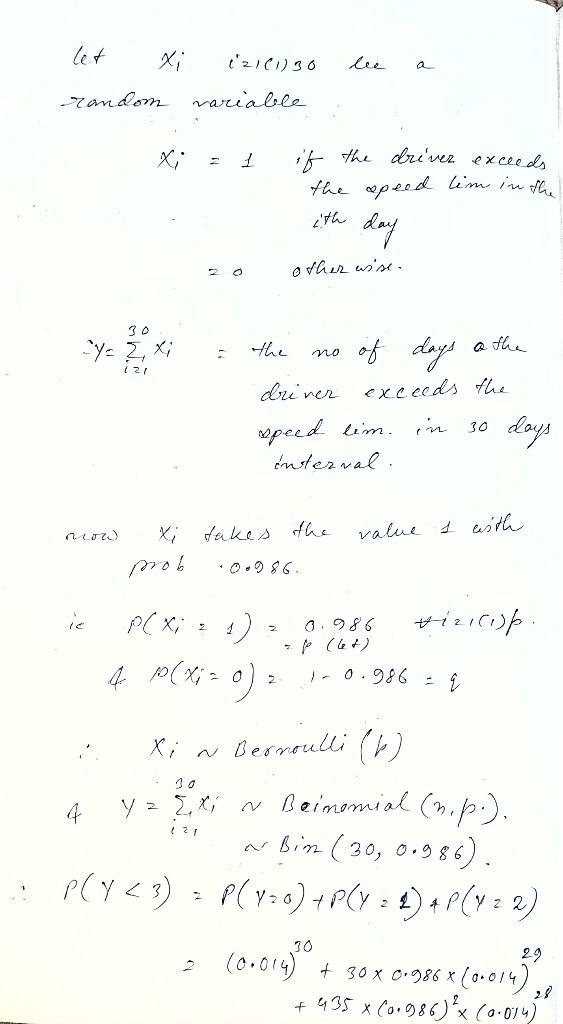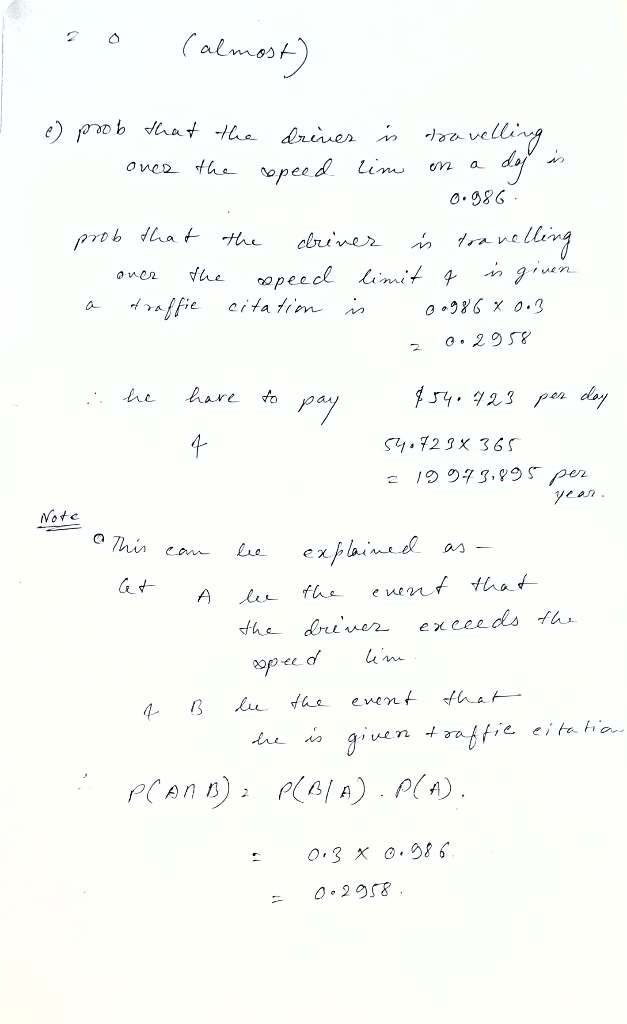# The daily travel speed of a driver on a highway segment (X) has mean 58 mph and coefficient of va...The daily travel speed of a driver on a highway segment (X) has mean 58 mph and coefficient of variation 0.42. The speed limit at this highway segment is 75 mph. Assuming that the probability density function of X is lognormal: a. What is the probability that the driver violates the speed limit on any given day? b. What is the probability that the driver exceeds the speed limit less than 3 times over a month? c. There is a 30% percent chance that a driver traveling over the speed limit is given a traffic citation at \$185 per citation. What is the expected cost of speeding ticket for the driver over a year (365 days)?